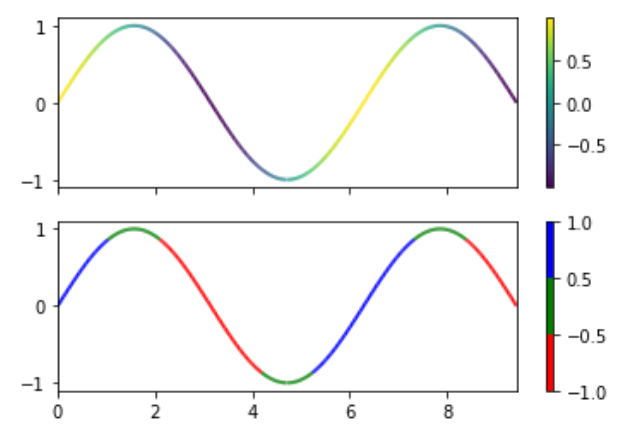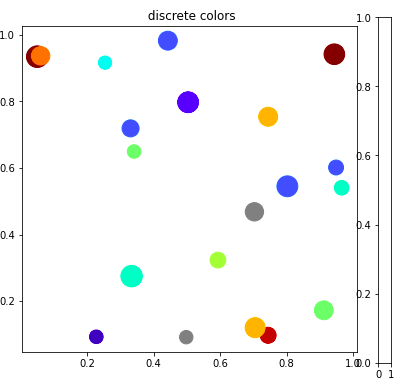Related Articles
Matplotlib.colors.BoundaryNorm class in Python
• Last Updated : 21 Apr, 2020

Matplotlib is an amazing visualization library in Python for 2D plots of arrays. Matplotlib is a multi-platform data visualization library built on NumPy arrays and designed to work with the broader SciPy stack.

## matplotlib.colors.BoundaryNorm

The matplotlib.colors.BoundaryNorm class belongs to the matplotlib.colors module. The matplotlib.colors module is used for converting color or numbers arguments to RGBA or RGB.This module is used for mapping numbers to colors or color specification conversion in a 1-D array of colors also known as colormap.

The matplotlib.colors.BoundaryNorm class is used to create a colormap based on discrete intervals. BoundaryNorm maps values to integers, unlike Normalize or LogNorm which does mapping to the interval of 0 to 1. Piecewise linear interpolation can be used for mapping to the o- interval, however, using integers is simpler and leads to the reduction of the number of conversions back and forth among integer and floating points.

Parameters:

1. boundaries: it is an array like object that monotonically increase sequence of boundaries
2. ncolor: It accepts an integer value that represents a number of colors in the colormap that will be used.
3. clip: It accepts a boolean value and is a optional parameter. If the clip is `True`, the values that are out of range and they are below the boundaries are mapped to 0, whereas if they are above the boundaries[-1] they are mapped to `ncolors-1`. If the clip is set to False, the out of range values and they are below the `boundaries` are mapped to -1, whereas if they are above boundaries[-1], they are mapped to ncolors. The `Colormap.__call__()` convert these into valid indices.

Note: The edges of bins are defined by boundaries and the data falling within the bins are mapped to the same color index. If the ncolors are not equal to the number of bins, linear interpolation is used to choose color for them.

Example 1:

 `import` `numpy as np``import` `matplotlib.pyplot as plt``from` `matplotlib.collections ``import` `LineCollection``from` `matplotlib.colors ``import` `ListedColormap, BoundaryNorm`` ` `a ``=` `np.linspace(``0``, ``3` `*` `np.pi, ``500``)``b ``=` `np.sin(a)``# this is the first derivative``dbda ``=` `np.cos(``0.5` `*` `(a[:``-``1``] ``+` `a[``1``:]))  `` ` `# Createing  line segments so``# to color them individually``points ``=` `np.array([a, b]).T.reshape(``-``1``, ``1``, ``2``)``set_of_segments ``=` `np.concatenate([points[:``-``1``],``                                  ``points[``1``:]],``                                 ``axis ``=` `1``)`` ` `figure, axes ``=` `plt.subplots(``2``, ``1``, ``                            ``sharex ``=` `True``, ``                            ``sharey ``=` `True``)`` ` `# Mapping the data points with``# continous norm``continous_norm ``=` `plt.Normalize(dbda.``min``(), ``                               ``dbda.``max``())`` ` `line_collection ``=` `LineCollection(set_of_segments,``                                 ``cmap ``=``'viridis'``,``                                 ``norm ``=` `continous_norm)`` ` `# Set the values used for ``# colormapping``line_collection.set_array(dbda)``line_collection.set_linewidth(``2``)``line ``=` `axes[``0``].add_collection(line_collection)``figure.colorbar(line, ax ``=` `axes[``0``])`` ` `# Use a boundary norm instead``cmap ``=` `ListedColormap([``'r'``, ``'g'``, ``'b'``])``boundary_norm ``=` `BoundaryNorm([``-``1``, ``-``0.5``, ``0.5``, ``1``],``                             ``cmap.N)`` ` `line_collection ``=` `LineCollection(set_of_segments, ``                                 ``cmap ``=` `cmap, ``                                 ``norm ``=` `boundary_norm)`` ` `line_collection.set_array(dbda)``line_collection.set_linewidth(``2``)``line ``=` `axes[``1``].add_collection(line_collection)``figure.colorbar(line, ax ``=` `axes[``1``])`` ` `axes[``0``].set_xlim(a.``min``(), a.``max``())``axes[``0``].set_ylim(``-``1.1``, ``1.1``)``plt.show()`

Output:Example 2:

 `import` `numpy as np``import` `matplotlib as mpl``import` `matplotlib.pylab as plt`` ` ` ` `# setup the plot``figure, axes ``=` `plt.subplots(``1``, ``1``, ``                            ``figsize``=``(``6``, ``6``))`` ` `# defining random data``x ``=` `np.random.rand(``20``)``y ``=` `np.random.rand(``20``)  ``tag ``=` `np.random.randint(``0``, ``20``, ``20``)``tag[``10``:``12``] ``=` `0`` ` `# defining the colormap``cmap ``=` `plt.cm.jet  `` ` `# extracting all colors``cmaplist ``=` `[cmap(i) ``for` `i ``in` `range``(cmap.N)]`` ` `# making first color entry  grey``cmaplist[``0``] ``=` `(.``5``, .``5``, .``5``, ``1.0``)`` ` `# new map``cmap ``=` `mpl.colors.LinearSegmentedColormap.from_list(``   ``'Custom cmap'``, cmaplist, cmap.N)`` ` `# defining the bins and norms``bounds ``=` `np.linspace(``0``, ``20``, ``21``)``norm ``=` `mpl.colors.BoundaryNorm(bounds,``                               ``cmap.N)`` ` `# the scatter``scat ``=` `axes.scatter(x, y, c``=``tag, ``                    ``s``=``np.random.randint(``100``,``                                        ``500``, ``                                        ``20``),``                    ``cmap``=``cmap, norm``=``norm)`` ` `# axes for the colorbar``ax2 ``=` `figure.add_axes([``0.95``, ``0.1``,``                       ``0.03``, ``0.8``])`` ` ` ` `axes.set_title(``' discrete colors'``)`

Output:Attention geek! Strengthen your foundations with the Python Programming Foundation Course and learn the basics.

To begin with, your interview preparations Enhance your Data Structures concepts with the Python DS Course. And to begin with your Machine Learning Journey, join the Machine Learning – Basic Level Course

My Personal Notes arrow_drop_up